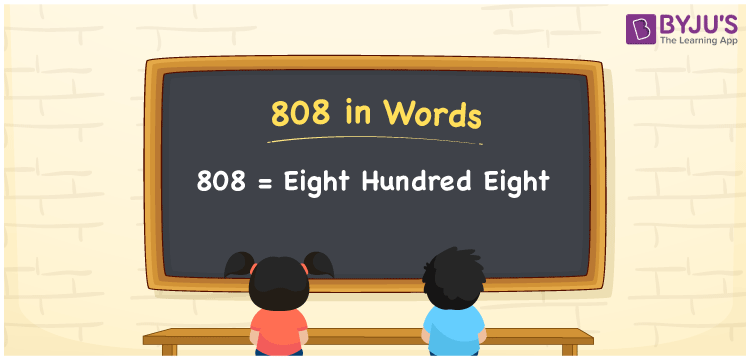# 808 in Words

The number 808 in words is written as “Eight hundred eight”. If you spent Rs. 808 on buying flowers, you can write, “I spent Rs. Eight hundred eight on buying flowers”. The number 808 is a natural number and it contains three-digits. Also, 808 is a cardinal number.

 808 in Words: Eight Hundred Eight. Eight Hundred Eight in Numerical Form: 808.

## 808 in English Words## How to Write 808 in Words?

Understand the place values of 808 using the below table and write the number 808 in words.

 Hundreds Tens Ones 8 0 8

The expanded form of 808 is as follows:

= 8 × Hundred + 0 × Ten + 8 × One

= 8 × 100 + 0 × 10 + 8 × 1

= 800 + 8

= 808

= Eight hundred eight

Hence, 808 in words is eight hundred eight.

808 in words – Eight hundred eight

Is 808 an odd number? – No

Is 808 an even number? – Yes

Is 808 a perfect square number? – No

Is 808 a perfect cube number? – No

Is 808 a prime number? – No

Is 808 a composite number? – Yes

## Frequently Asked Questions on 808 in Words

Q1

### Write 808 in English words.

808 in words is eight hundred eight.

Q2

### Simplify 800 + 8, and express it in words.

Simplifying 800 + 8, we get 808. Hence, 808 in words is eight hundred eight.

Q3

### Is 808 an even number?

Yes, 808 is an even number.